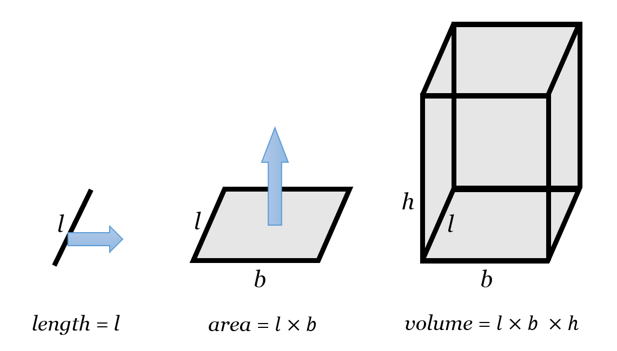Open in App
Not now

# What is the formula for volume of cuboid?

• Difficulty Level : Basic
• Last Updated : 27 Sep, 2021

Mensuration is a component of Geometry that deals with defining shapes/solids by calculations on their dimensions. It describes geometrical entities by their physical properties like length, different types of surface area, perimeter, and volume. Like any other domain falling under Mathematics, mensuration too has abundant real-life applications ranging from finding the length of the earth’s equator to measuring the fence length around a piece of land.

### Cuboid

A ‘cuboid’ is the first visual that would occur in your mind when you imagine a 3D rectangle! In real life, cuboids can be seen in so many places, from books to cupboards to laptops, etc. has forms of cuboids. Let’s look at some of the important characteristics of cuboids. A cube is a special cuboid in which all the 3 dimensions – length, breadth, and height are equal.

• A cuboid has 6 faces.
• Every corner of a cuboid is a right angle (90°)
• Every face is a rectangle.

### What is the formula of volume of the cuboid?

A cuboid of length l, breadth b, and height h can be visualized as follows:

• A line of length l, if stretched till a distance b in a perpendicular direction, would form a rectangular surface that would occupy the l×b area.
• The same surface if stretched in a perpendicular direction (for eg, upwards), would form a cuboid that would occupy l×b×h space.

• Unit of volume is unit3 (usually, cm3 or m3)Visualization

### Sample Problems

Question 1: What is the volume of a cuboid with length 20 cm, breadth 12 cm, and height 5 cm?

Solution:

Volume = l×b×h

= 20×12×5

= 1200 cm3

Question 2: What is the volume of a cuboid whose top face is a square of area 16 cm2 and height is 8cm?

Solution:

As the top face is square with a 16cm2 area, we get l × b = 16.

⇒ Volume = (l × b) × h

= 16 × 8

= 128 cm3

Question 3: What is the volume of a wall that has a length of 10 m, width 20 cm, and height of 2 m?

Solution:

Volume = l×b×h

= 10m × 20cm × 2m

= 10m × 0.2m × 2m

= 4 m3

My Personal Notes arrow_drop_up